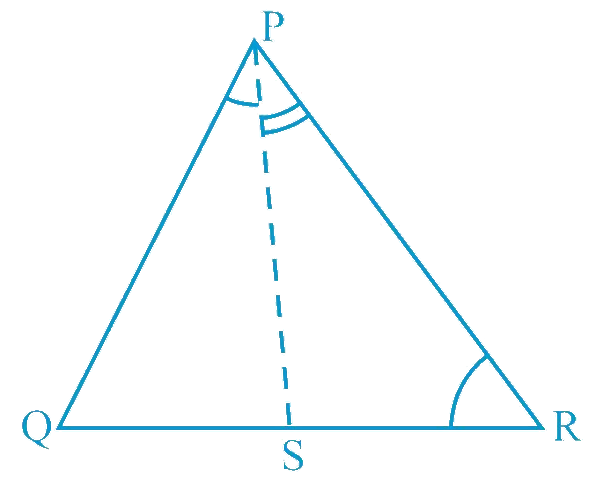# Ex.7.4 Q5 Triangles Solution - NCERT Maths Class 9

Go back to  'Ex.7.4'

## Question

In the given figure, $$PR \gt PQ$$ and $$PS$$

bisects $$\angle QPR$$. Prove that

$$\angle PSR \gt \angle PSQ$$.

Video Solution
Triangles
Ex 7.4 | Question 5

## Text Solution

What is Known?

$$\text{PR PQ}$$ and PS bisects $$\angle \text{QPR}$$

To prove:

$\angle \text{PSR }>\angle \text{PSQ}\text{.}$

Reasoning:

We can use exterior angle sum property to find the required inequality.Steps:

As $$PR \gt PQ$$,

\begin{align} & \angle PQR \gt \angle PRQ\\&\left(\begin{array}{} \text {Angle opposite to larger}\\\text{ side is larger}) \ldots (1)\end{array}\right) \\\\ & PS \text { is the bisector of } \angle QPR \\ & \angle QPS = \angle RPS \ldots (2) \\\\ & \angle PSR \text{ is the exterior angle of } PQS \\ & \angle PSR = \angle PQR + \angle QPS \ldots (3)\\\\ & \angle PSQ \text{ is the exterior angle of } PRS \\ & \angle PSQ = \angle PRQ + \angle RPS \ldots (4)\\ \end{align}

Adding Equations ($$1$$) and ($$2$$), we obtain

\begin{align} &\angle PQR + \angle QPS \gt \angle PRQ + \angle RPS \\ & \angle PSR \gt \angle PSQ \\& \begin{bmatrix}\text{Using the values of}\\ \text{Equations (2),(3) and (4)}\end{bmatrix}\end{align}

Video Solution
Triangles
Ex 7.4 | Question 5

Learn from the best math teachers and top your exams

• Live one on one classroom and doubt clearing
• Practice worksheets in and after class for conceptual clarity
• Personalized curriculum to keep up with school